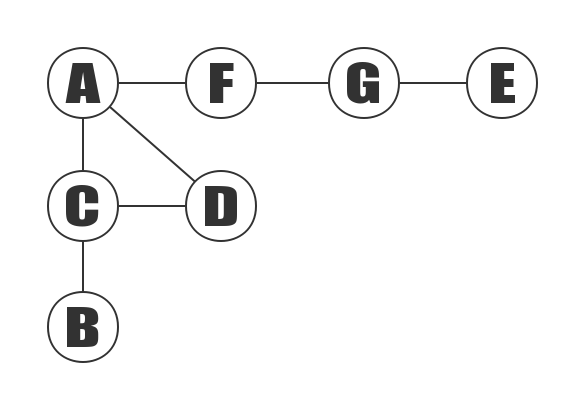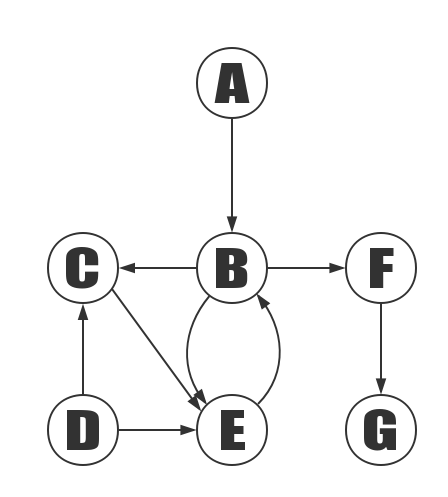Adjacency matrix undirected graph refers to an undirected graph represented by an adjacency matrix.The above graph contains 7 vertices of A, B, C, D, E, F, G, and it also contains, , , , , , , in total 7 edges. Since this is an undirected graph, the edge and the edge are the same edges. The table of edges is listed in alphabetical order.

N/A A B C D E F G
A 0 0 1 1 0 1 0
B 0 0 1 0 0 0 0
C 1 1 0 1 0 0 0
D 1 0 1 0 0 0 0
E 0 0 0 0 0 0 1
F 1 0 0 0 0 0 1
G 0 0 0 0 1 1 0

The matrix above is a schematic of the adjacency matrix in memory. A[i][j] = 1 means that from the ith vertex to the jth vertex is a edge. A[i][j] = 0 indicates that they are not adjacent points.

### C++ Implementation

Adjacency matrix undirected graph refers to an directed graph represented by an adjacency matrix.The above graph contains 7 vertices of A, B, C, D, E, F, G, and it also contains , , , , , , , , , in total 7 edges.

N/A A B C D E F G
A 0 0 1 0 0 0 0
B 0 0 1 0 1 1 0
C 0 0 0 0 1 0 0
D 0 0 1 0 0 0 0
E 0 1 0 1 0 0 1
F 0 0 0 0 0 0 1
G 0 0 0 0 0 0 0

The matrix above is a schematic of the adjacency matrix in memory. A[i][j] = 1 means that the ith vertex and the jth vertex are adjacent points. A[i][j] = 0 indicates that not a edge.

Share